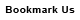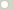# dyne centimeter (Torque, Metric)

## 1 dyne centimeter is equal to:Metric newton meter 1e-07 newton centimeter 1e-05 dyne meter 0.01 kilogram-force meter 1.0197162e-08 kilogram-force centimeter 1.0197162e-06 gram-force meter 1.0197162e-05 gram-force centimeter 0.0010197162 British and U.S. long ton-force foot 3.2926882e-11 short ton-force foot 3.6878107e-11 pound-force foot 7.3756215e-08 pound-force inch 8.8507458e-07 ounce-force inch 1.4161193e-05ConversionsAreaCapacity and VolumeCircular measureComputer storageCurrencyDistance and LengthEnergy and WorkFuel ConsumptionMass and WeightPowerPressureSpeedTemperatureTimeTorqueSearchLink to us